# pylops.FirstDerivative¶

class pylops.FirstDerivative(N, dims=None, dir=0, sampling=1.0, edge=False, dtype='float64')[source]

First derivative.

Apply second-order centered first derivative.

Parameters: N : int Number of samples in model. dims : tuple, optional Number of samples for each dimension (None if only one dimension is available) dir : int, optional Direction along which smoothing is applied. sampling : float, optional Sampling step dx. edge : bool, optional Use reduced order derivative at edges (True) or ignore them (False) dtype : str, optional Type of elements in input array.

Notes

The FirstDerivative operator applies a first derivative to any chosen direction of a multi-dimensional array.

For simplicity, given a one dimensional array, the second-order centered first derivative is:

$y[i] = (0.5x[i+1] - 0.5x[i-1]) / dx$
Attributes: shape : tuple Operator shape explicit : bool Operator contains a matrix that can be solved explicitly (True) or not (False)

Methods

 __init__(self, N[, dims, dir, sampling, …]) Initialize this LinearOperator. adjoint(self) Hermitian adjoint. cond(self, \*\*kwargs_eig) Condition number of linear operator. conj(self) Complex conjugate operator div(self, y[, niter]) Solve the linear problem $$\mathbf{y}=\mathbf{A}\mathbf{x}$$. dot(self, x) Matrix-matrix or matrix-vector multiplication. eigs(self[, neigs, symmetric, niter]) Most significant eigenvalues of linear operator. matmat(self, X) Matrix-matrix multiplication. matvec(self, x) Matrix-vector multiplication. rmatvec(self, x) Adjoint matrix-vector multiplication. transpose(self) Transpose this linear operator.

## Examples using pylops.FirstDerivative¶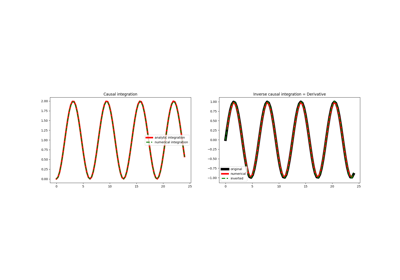Causal Integration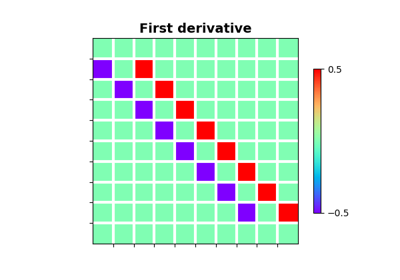Derivatives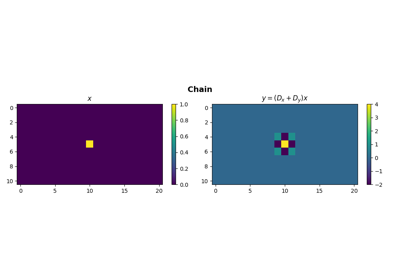Operators concatenation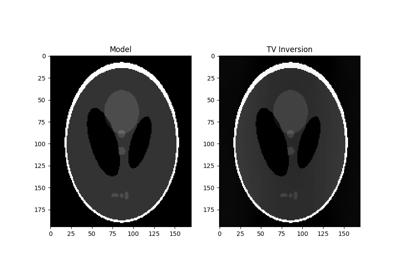Total Variation (TV) Regularization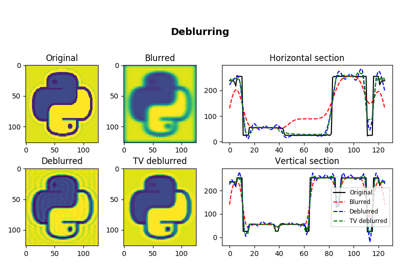04. Image deblurring